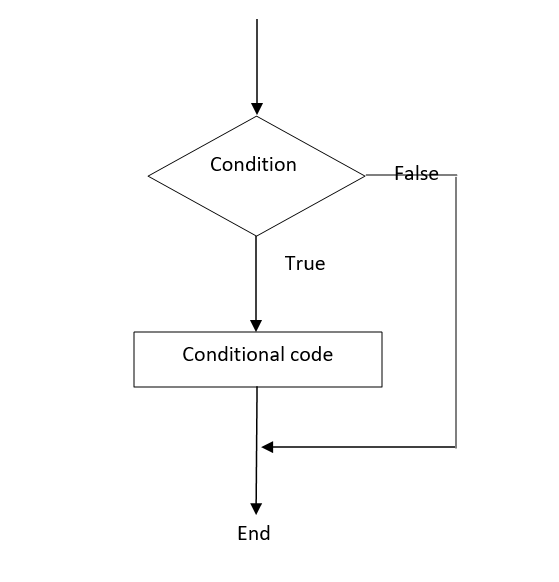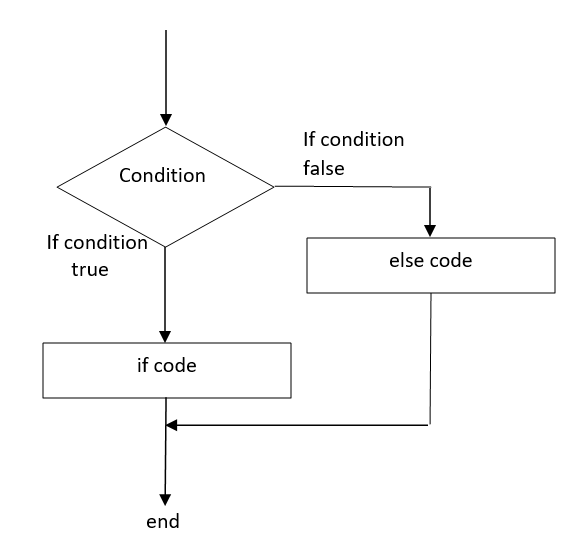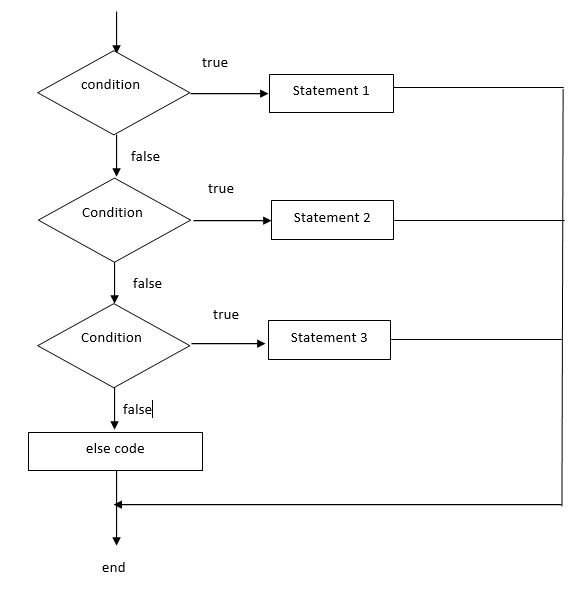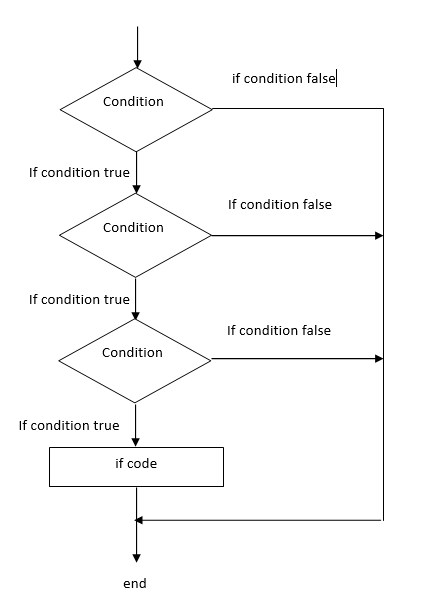# Swift – Decision Making Statements

• Last Updated : 20 Dec, 2021

Decision-making statements are those statements in which the compiler needs to make decisions on the given conditions. If the given condition is satisfied then the set of statements is executed otherwise some other set of statements are executed. These statements always return a boolean value that is either true or false. If true then the if block is executed. Otherwise else (or) else if block is executed. Swift supports five different decision-making statements and they are :

1. Simple if
2. if-else
3. if – else if – else
4. Nested if
5. Switch

### Simple if statement

In Swift, a simple if works based on condition. If the given condition is satisfied, then the statements inside the if block is executed. If the given condition is false then the control return from the if statement and executes the statement present after the if statement block.

Syntax:

if condition

{

// statements

}

Flow diagram:Example:

## Swift

 `// Swift program to illustrate the if statement`` ` `// Declaring values into variables``let` `a = 10``let` `b = 20`` ` `// Checking condition through if ``if` `(a < b)``{``    ``// If condition is true then the below``    ``// statement is executed ``    ``print``(``"Welcome to GeeksforGeeks"``)``}`

Output:

`Welcome to GeeksforGeeks`

### if – else Statement

In Swift, if – else statement is just like other programming languages. If the condition is satisfied or true then the statements in the if block are executed. If the condition is not satisfied then the statements in the else block are executed.

Syntax:

if condition

{

// Statements

}

else

{

// Statements

}

Flow diagram:Example:

## Swift

 `// Swift program to illustrate the if-else statement`` ` `// Declaring values to variables``let` `a = 10``let` `b = 20`` ` `// Checking if condition``if` `(a > b)``{``    ``// If condition true then the following``    ``// statement is executed``    ``print``(``"a is largest number"``)``}``else``{``    ``// If the condition not satistied then``    ``// the below statement is executed ``    ``print``(``"b is largest number"``)``}`

Output:

`b is the largest number`

### if-else if-else Statement

Generally else doesn’t have any condition checking part so when if condition fails then else part will be executed automatically. But using else if condition we can write a condition in else part also. We can write n number of else if conditions. So, it is called as if-else if ladder.

Syntax:

if condition

{

// Statements

}

else if condition

{

// Statements

}

….

else

{

// Statements

}

Flow diagram:Example:

## Swift

 `// Swift program to illustrate the ``// if-else if-else statement`` ` `// Declaring values into variables``let` `a = 10``let` `b = 25``let` `c` `= 20`` ` `// Checking whether a is biggest``if` `a > b && a > ``c``{``    ``// If true printing message``    ``print``(``"a is biggest number"``)``}`` ` `// If the above condition false ``// then checking b is biggest``else` `if` `b > ``c``{``    ``// If true printing message``    ``print``(``"b is biggest number"``)``}`` ` `// If the above condition false printing ``// the remaining number is biggest``else``{``    ``print``(``"c is biggest number"``)``}`

Output:

`b is biggest number`

### Nested if Statement

Nested means within. Nested if means if within if condition. Generally in if-else, if condition satisfied then the statements under if are executed. But in nested if, again condition is checked. Like that in single if condition we can write many if conditions. The statements are executed only after all the if conditions are satisfied. Otherwise else condition statements are executed.

Syntax:

if condition

{

if condition

{

if condition

{

……

//statements

}

}

}

else

{

//statements

}

Flow diagram:Example:

## Swift

 `// Swift program to illustrate the ``// Nested if statement`` ` `// Declaring values into variables``let` `a = 10``let` `b = 20`` ` `// Nested if statement``if` `a == 10``{``    ``if` `b == 20``    ``{``        ``print``(``"geeks"``)``    ``}``}``else``{``    ``print``(``"geeksforgeeks"``)``}`

Output:

`geeks`

### switch Statement

A switch is also like if – else if ladder we can write n number of else if conditions. Likewise in the switch statement also we can write n number of cases. if-else if ladder works automatically based on conditions. But the switch statement works like a switch. Based on the choice the desired case is executed. For example, we have chosen 2, then case 2 will be executed. And there is a default case, if all the cases are failed then the default case is executed. It is not mandatory to write a default case, it’s an optional case. And we write a break statement to stop controls to fall through the next subsequent cases.

Syntax:

switch(expression)

{

case constant-expression :

statement(s);

break;

case constant-expression :

statement(s);

break;

default : optional

statement(s);

}

Example:

## Swift

 `// Swift program to illustrate the ``// switch statement`` ` `// Declaring values into variables``let` `abc = 10`` ` `// Switch statement. Here we have 4 cases and ``// our choice is 10. There is no case 10``// so, default case is executed``switch` `abc``{``    ``case` `1:``        ``print``(``"This is case 1"``)``        ``break``;``    ``case` `2:``        ``print``(``"This is case 2"``)``        ``break``;``    ``case` `3:``        ``print``(``"This is case 3"``)``        ``break``;``    ``case` `4:``        ``print``(``"This is case 4"``)``        ``break``;``    ``default``:``        ``print``(``"Example of switch statement using break"``)``}`

Output:

`Example of switch statement using break`

My Personal Notes arrow_drop_up